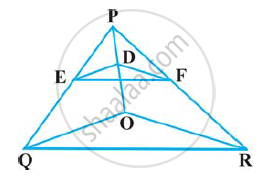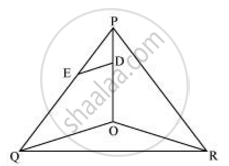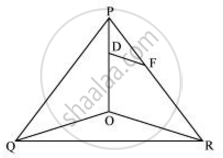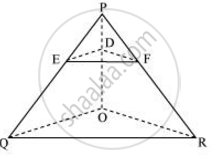# In the following figure, DE || OQ and DF || OR, show that EF || QR - Mathematics

In the following figure, DE || OQ and DF || OR, show that EF || QR#### SolutionIn Δ POQ, DE || OQ

:. (PE)/(EQ) = (PD)/(DO) .... (Basic Proportionality theorem)  (i)In ΔPOR, DF || OR

:. (PF)/(FR) = (PD)/(DO) (Basic Proportionality theorem)  (ii)

From i and ii we obtain

(PE)/(EQ) = (PF)/(FR)

:. EF || QR (Converse of basic proportionally theorem)Concept: Similarity of Triangles
Is there an error in this question or solution?

#### APPEARS IN

NCERT Class 10 Maths
Chapter 6 Triangles
Exercise 6.2 | Q 5 | Page 129